Students must start practicing the questions from CBSE Sample Papers for Class 10 Maths with Solutions Set 9 are designed as per the revised syllabus.

## CBSE Sample Papers for Class 10 Maths Standard Set 9 with Solutions

Time Allowed: 3 Hours
Maximum Marks: 80

General Instructions:

1. This Question Paper has 5 Sections A-E.
2. Section A has 20 MCQs carrying 1 mark each
3. Section B has 5 questions carrying 02 marks each.
4. Section C has 6 questions carrying 03 marks each.
5. Section D has 4 questions carrying 05 marks each.
6. Section E has 3 case based integrated units of assessment (04 marks each) with sub-parts of the values of 1, 1 and 2 marks each respectively.
7. All Questions are compulsory. However, an internal choice in 2 Qs of 5 marks, 2 Qs of 3 marks and 2 Questions of 2 marks has been provided. An internal choice has been provided in the 2 marks questions of Section E
8. Draw neat figures wherever required. Take π =22/7 wherever required if not stated.

Section – A
(Section A consists of 20 questions of 1 mark each.)

Question 1.
If sin a and cos a are the roots of the equation ax2 + fax + c = 0, then fa2 is equal to:
(a) a2 – 2ac
(b) a2 + 2ac
(c) a2 – ac
(d) a2 + ac
(b) a2 + 2ac

Explanation:
Given, sin a and cos a are the roots of the equation ax2 + bx + c = 0
⇒ sin α + cos α = $$\frac{-b}{a}$$
and sin α cos α =$$\frac{c}{a}$$
(sin α + cos α)2 = sin2 α + cos2 α + 2 sin α cos α
⇒ $$\frac{b^2}{a^2}=1+\frac{2 c}{a}$$
⇒ b2 = a2 + 2ac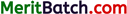Question 2.
In a rectangle OABC the length of its diagonal is, if its three vertices are O (0, 0), A (0, 3) and B(5, 0):
(a) 5 units
(b) $$\sqrt{34}$$ units
(c) 4 units
(d) 3 units
(b) $$\sqrt{34}$$ units

Explanation:
Here, diagonal is OC as per the figure.
Length of diagonal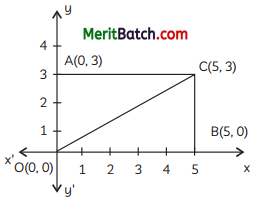OC = $$\sqrt{(5-0)^2 + (3-0)^2}$$
= $$\sqrt{25+9}$$
= $$\sqrt{34}$$ units

Question 3.
If radii of two concentric circles are 8 cm and 10 cm, then the length of each chord of one circle which is tangent to the other circle is:
(a) 1 cm
(b) 3 cm
(c) 12 cm
(d) 9 cm
(c) 12 cm

Explanation:
Here, ABC is a chord in bigger circle and tangent to smaller circle.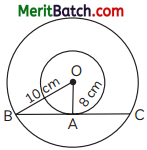∴ ∠OBA = 90°
(As tangent is ⊥r to radius at the point of contact)
∴ OB will bisect chord AC as ⊥r from the centre bisect the chord.
∴ In DOBA,
OA2 = OB2 + AB2
⇒ 102 = 82 + AB2
⇒ AB2 = 100 – 64 = 36
⇒ AB = 6 cm
Hence, AC = 2 AB = 2 × 6 = 12 cm

Question 4.
For the following distribution:

 Marks obtained Number of students Below 10 3 Below 20 12 Below 30 27 Below 40 57 Below 50 75 Below 60 80

The median class is:
(a) 10 – 20
(b) 20 – 30
(c) 30 – 40
(d) 40-50
c) 30-40

Explanation:
Here, N = 80
$$\frac{N}{2}$$ = 40Question 5.
In an AP, if a = 1, an = 17 and Sn = 216, then n is:
(a) 19
(b) 21
(c) 24
(d) 42
(c) 24

Explanation:
Given: a = 1, an = 17 and Sn = 216
Now, a + (n – 1) d = 17
1 + (n – 1) × d = 17
⇒ (n – 1)d = 16 ……(i)
and Sn = 216
$$\frac{n}{2}$$[2a + (n – 1) d] = 216
⇒ $$\frac{n}{2}$$ [2 + 16] = 216
⇒ $$\frac{n}{2}$$ × 18 = 216
⇒ n = 24

Question 6.
The zeroes of the quadratic polynomial x2 + kx + k, where k ≠ 0.
(a) cannot both be positive
(b) cannot both be negative
(c) are always equal
(d) are always equal
(a) cannot both be positive

Explanation:
Given : p(x) = x2 + kx + k

Sum of zeroes = –$$\frac{b}{a}$$ = –$$\frac{k}{a}$$ = -k
Product of zeroes = $$\frac{c}{a}$$ = k
If k is negative
Sum is possible, product is negative
∴ One zero will be positive, one will be negative.
If k is positive.
Sum is negative but product is positive.
∴ Both zeroes are negative
So, in both cases, both zeroes cannot be posi¬tive.

Question 7.
The pair of linear equations: 3x – y + 9 = 0 and 6x – 2y + 10 = 0 represents two lines which are:
(a) intersecting at exactly one point
(b) coincident
(c) parallel
(d) cannot be determined 1
(c) parallel

Explanation:
Given pair of linear equations are:
3x – y + 9 = 0
6x – 2y + 10 = 0
On comparing the given equations by:
a1x + b1y + c1 = 0
and a2x + b2y + c2 = 0
∴ a1 = 3, b1 = – 1, c1 = 9
and a2 = 2, b2 = -2, c2 = 10
∴ $$\frac{a_1}{a_2}=\frac{3}{6}=\frac{1}{2}, \frac{b_1}{b_2}=\frac{-1}{-2}=\frac{1}{2}, \frac{c_1}{c_2}=\frac{9}{10}$$
∴ $$\frac{a_1}{a_2}=\frac{b_1}{b_2} \neq \frac{c_1}{c_2}$$
Hence, the Lines are parallel 8.Question 8.
The distance of the point P(-3, -4) from the x-axis is:
(a) 5
(b) -3
(c) 3
(d) 4
(d) 4

Explanation:
Perpendicular distance of a point from x-axis = |y – coordinate of that point|
∴ For point (-3, -4)
Required distance = |-4| = 4 units

Question 9.
A hollow cube of internal edge 22 cm is filled with spherical marbles of diameter 0.5 cm and it is assumed that $$\frac{1}{8}$$ space of the cube remains unfilled. How many marbles are there in the cube?
(a) 1,42,296
(b) 1,42,396
(c) 1,42,496
(d) 1,42,596
(a) 1,42,296

Explanation:
Side of cube, a = 22 cm
Diameter of spherical marbles, d = 0.5 cm
Radius of spherical marbles, r = 05 cm
Volume of cube = a3 = (22)3
$$\frac{1}{8}$$th of cube is unfilled, then volume of cube 8
unfilled = $$\frac{1}{8}$$ × 22 × 22 × 22
= 11 × 11 × 11 = (11)3
Volume of cube filled = (22)3 – (11)3
Let number of spherical marbles be x.
then, n × $$\frac{4}{3}$$πr3 = (22)3 – (11)3
n × $$\frac{4}{3}$$ × $$\frac{22}{7}$$ × $$\frac{0.5}{2}$$ × $$\frac{0.5}{2}$$ × $$\frac{0.5}{2}$$ = 10648 – 1331
n = $$\frac{9317 × 8 × 21}{88 × 0.5 × 0.5 × 0.5}$$
= 1,42,296

Question 10.
If 4 tan θ = 3, then the value of $$\left(\frac{4 \sin \theta-\cos \theta}{4 \sin \theta+\cos \theta}\right)$$ is :
(a) 0
(b) $$\frac{1}{4}$$
(c) $$\frac{1}{2}$$
(d) $$\frac{3}{4}$$
(c) $$\frac{1}{2}$$

Explanation:
4 tan θ = 3Question 11.
All the three face cards of spades are removed from a pack of 52 playing cards. A card is drawn at random from the remaining pack. The probability that the card drawn is black queen, is:
(a) $$\frac{1}{49}$$
(b) $$\frac{3}{49}$$
(c) $$\frac{1}{13}$$
(d) $$\frac{3}{13}$$
(a) $$\frac{1}{49}$$

Explanation: Remaining cards in a pack = 52 -3 = 49
Now, only 1 black queen of club will be left.
P(black queen card) = $$\frac{1}{49}$$

Question 12.
What is the relation between the area of a circle and square, if the circumference of a circle and the perimeter of a square are equal.
(a) Area of the circle = Area of the square
(b) Area of the circle < Area of the square (c) Area of the circle > Area of the square
(d) Nothing can be said about the relation between the areas of the circle and the square.
(c) Area of the circle > Area of the square

Explanation:
Let, the radius of circle be ‘r’ and side of square be ‘a’.
Then, 2πr = 4a
or πr = 2a
or a = $$\frac{πr}{2}$$ = $$\frac{11}{7}$$r
Now, area of circle = πr2 = $$\frac{22}{7}$$r2 = $$\frac{22×7}{7×7}$$
= $$\frac{154}{49}$$r2
area of square = a2 = ($$\frac{11}{7}$$)2r2
= $$\frac{121}{49}$$r2
Area of circle > Area of square.

Question 13.
The least number that is divisible by all the numbers from 1 to 10 (both exclusive) is:
(a) 10
(b) 504
(c) 100
(d) 5040
(d) 5040

Explanation:
The numbers are 2, 3, 4, 5, 6, 7, 8, 9Question 14.
If the ratio of a cone’s radius to a cylinder’s radius is 3 : 5, then the ratio of their heights is:
(a) $$\frac{25}{3}$$
(b) $$\frac{28}{3}$$
(c) $$\frac{23}{3}$$
(d) 7
(a) $$\frac{25}{3}$$

Explanation:
Let r1 and h1 be the radius and height of the cone and r2 and h2 be the radius and height of the cylinder.

Volume of cone = $$\frac{1}{3} r_1^2 h_1$$
Volume of cylinder = $$\pi r_2^2 h_2$$
It is given that the volume of cone is equal to the volume of cylinder.Question 15.
If k, 2k – 1 and 2k + 1 are three consecutive terms of an AP, then the value of k is:
(a) 2
(b) 3
(c) -3
(d) 5
(b) 3

Explanation: Since, the terms k, 2k – 1, 2k + 1 are in A.P.
We know that,
2b = a + c
∴ 2(2k – 1) = k + 2k + 1
⇒ 4k – 2 = 3k + 1
⇒ 4k – 3k = 3
⇒ k = 3

Question 16.
A largest triangle is inscribed in a semi¬circle of radius a units, then its area is: (1)
(a) a2 sq units
(b) 2a2 sq units
(c) $$\frac{1}{2}$$a2 sq units
(d) $$\sqrt{2}$$a2 sq units
(a) a2 sq units

Explanation:AB is the diameter of the semi-circle.
∴ AB = 2a
Side of the triangle AB = 2a,
OC = a
∠ACB = 90°
(as angle in a semi-circle)
area(largest DABC) = $$\frac{1}{2}$$ × AB × OC
= $$\frac{1}{2}$$ × 2a × a = a2 sq units

Question 17.
The pair of linear equations: -3x – 6y = 5 and x + 2y = 1 have:
(a) a unique solution
(b) infinitely many solutions
(c) no solution
(d) exactly two solutions
(c) no solution

Explanation:
Given pair of linear equations are:
-3x – 6y = 5
or -3x -6y -5 = 0
and x + 2y = 1
or x + 2y – 1 = 0
Here, a1 = -3, b1 = -6, c1 = -5
and a2 = 1, b2 = 2, c2 = -1
∴ $$\frac{a_1}{a_2}=\frac{-3}{1}, \frac{b_1}{b_2}=\frac{-6}{2}=\frac{-3}{1}, \frac{c_1}{c_2}=\frac{-5}{-1}=\frac{5}{1}$$
∴ $$\frac{a_1}{a_2}=\frac{b_1}{b_2} \neq \frac{c_1}{c_2}$$
Hence, the given pair of equations has no solution.Question 18.
The probability of picking a rotten apple from lot of 400 eggs is 0.035. The number of rotten apples in the lot is:
(a) 7
(b) 14
(c) 21
(d) 28
(b) 14

Explanation:
Total no. of apples = 400
Probability of getting a rotten apple = 0.035
∴ \begin{aligned} P(\text { rotten apple }) &=\frac{\text { No.of rotten apples }}{\text { Total no. of apples }} \\ 0.035 &=\frac{\text { No.of rotten apples }}{400} \end{aligned}
∴ No. of rotten apples = 400 × 0.035 = 14

DIRECTION: In the question number 19 and 20, a statement of assertion (A) is followed by a statement of reason (R).
Choose the correct option as:
(a) Both assertion (A) and reason (R) are true and reason (R) is the correct explanation of assertion (A)
(b) Both assertion (A) and reason (R) are true and reason (R) is not the correct explanation of assertion (A)
(c) Assertion (A) is true but reason (R) is false.
(d) Assertion (A) is false but reason (R) is true.

Question 19.
Statement A (Assertion): cos2 A + sin2 A = 1 is a trigonometric identity.
Statement R (Reason): An equation involving trigono-metric ratios of an angle is called trigonometric identity, if it is true for all values of the angles involved.
(a) Both assertion (A) and reason (R) are true and reason (R) is the correct explanation of assertion (A)

Explanation:
We have, cos2 A + sin2 A = 1 Put A = 45°,
we get cos2 45° + sin2 45° = $$\left(\frac{1}{\sqrt{2}}\right)^2+\left(\frac{1}{\sqrt{2}}\right)^2=\frac{1}{2}+\frac{1}{2}=1$$
This is a trigonometric identity.

Question 20.
Statement A (Assertion): In a circular drum of radius 4.2 m and height 3.5 m, the number of full bags of wheat can be emptied, if the space required for wheat in each bag is 2.1 cu. m, is 92 bags nearly.
Statement R (Reason): Volume of circular drum is $$\frac{1}{2}$$ πr2h where r is radius and h is height of drum.
2
(c) Assertion (A) is true but reason (R) is false.

Explanation:
Radius of circular drum, r = 4.2 m
Height of circular drum, h = 3.5 m
Volume of circular drum
= πr2h
= $$\frac{22}{7}$$ × 4.2 × 4.2 × 3.5 m
Space occupied by 1 wheat bag = 2.1 m3
Number of wheat bags = $$\frac{\text { Volume of drum }}{\text { Space occupied by } 1 \mathrm{bag}}$$Section – B
(Section B consists of 5 questions of 2 marks each.)

Question 21.
In an AP, the sequence defined by an = 4n + 5. Find its common difference.
OR
After finding the zeroes of the quadratic polynomial p(x) = 6x2 – 13x + 6, verify the relation between its zeroes and its coefficients.
an = 4n + 5
n = 1, 2, 3, 4
a1 = 4 × 1 + 5 = 9
a2 = 4 × 2 + 5 = 13
a3 = 4 × 3 + 5 = 17
a4 = 4 × 4 + 5 = 21
a4 = a3 = 21 – 17 = 4
a2 – a1 = a3 – a2 = a4 – a3
Hence, it forms an AP with common difference 4.

OR
Given, polynomial is:
6x2 – 13x + 6 = 0
⇒ 6x2 – 9x – 4x + 6 = 0
(on splitting the middle term)
⇒ 3x (2x – 3) – 2 (2x – 3) = 0
⇒ (2x – 3) (3x – 2) = 0
∴ x = $$\frac{3}{2}$$ and x = $$\frac{2}{3}$$

In the given polynomial,
Sum of zeroes = $$\frac{-b}{a}$$ = $$\frac{-(-13)}{6}$$ = $$\frac{13}{6}$$
Sum of zeroes = $$\frac{3}{2}+\frac{2}{3}=\frac{9+4}{6}=\frac{13}{6}$$
They are equal.

In the given polynomial,
Product of zeroes = $$\frac{6}{6}$$ = 1
and Product of zeroes = $$\frac{3}{2} \times \frac{2}{3}$$ = 1
They are equal.
Hence, the relation is verified.

Question 22.
ln a ∆ABC right-angled at B, if AB: AC = 1: $$\sqrt{2}$$, then find the value of $$\frac{2 tan A}{1 + tan^2 A}$$
Given: a right angled DABC, right angled at B. C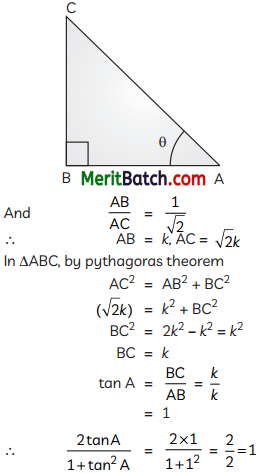Question 23.
Evaluate greatest number which divides 245 and 1029 and leaves the 5 as remainder in each case. 2
The given numbers are 245 and 1029
Since, the remainder is 5 in both cases,
So we have to subtract 5 from each number
245 – 5 = 240
1029 – 5 = 1024
Now, find the HCF of 240 and 1024.So, HCF = 2 × 2 × 2 × 2 = 16
Hence, the required greatest number is 16

Question 24.
An ice cream cone has a hemispherical top. If the height of the conical portion is 9 cm and base radius is 2.5 cm, determine the volume of ice-cream contained in the ice cream cone.
OR
A student recorded how many cars passed through a certain location on a road for 100 periods, each lasting three minutes, and summarised the data in the table below. Find the mean of the following table.

 Number of cars 0-10 10-20 20-30 30-40 40-50 Frequency 7 10 15 8 10

Base radius of cone = Base radius of hemisphere, r = 2.5 cm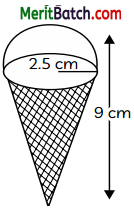Height of the cone, h = 9 cm
Volume of ice-cream in cone = volume of the cone + volume of hemisphere
=$$\frac{1}{3}$$πr2h + $$\frac{2}{3}$$πr3
=$$\frac{1}{3}$$πr2(h+r)
= $$\frac{22}{7}$$ ×$$\frac{2.5}{3}$$ × 2.5 (9 + 2.5)
= $$\frac{22}{21}$$ × 6.25 × 11.5
= 75.29
= 75.3 cm³
Hence the required volume is 75.3 cm³
OR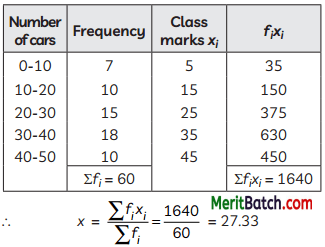Question 25.
In two concentric circles, if a chord AB of the outer circle is a tangent to the inner circle at P, then prove that P is the mid-point of AB.
Given: Two concentric circles with centre O.
AB is a chord in bigger circle and tangent at point P in the inner circle.
To Prove : AP = PB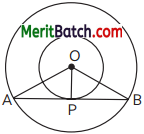Proof : Since, tangent to a circle is ⊥ to the radius at the point of contact.
∠APO = 90°
Now, for bigger circle, the ⊥ from the centre bisects the chord.
AP = PB
Hence, Proved.

Section – C
(Section C consists of 6 questions of 3 marks each.)

Question 26.
The zeroes of a quadratic polynomial are in the ratio 2 : 3 and their sum is 27. Find the product of these zeroes.
Let, the zeroes of quadratic polynomial be 2x and 3x.
Given, their sum is 27 \ 2x + 3x = 27
5x = 27

Let, the zeroes of quadratic pol.ynomiaL be 2x and 3x.
Given, their sum is 27
∴ 2x + 3x = 27
5x = 27
x = $$\frac{27}{5}$$

∴ Zeroes are 2 × $$\frac{27}{5}$$ and 3 × $$\frac{27}{5}$$

Consider, α = $$\frac{54}{5}$$ and ß = $$\frac{81}{5}$$
Then, product of zeroes
αß = $$\frac{54}{5}$$ × $$\frac{81}{5}$$
= $$\frac{4374}{25}$$

Question 27.
If cosec θ + cot θ = λ, then show that (λ2 + 1) cos θ = λ2 – 1
Given cosec θ + cot θ = λ,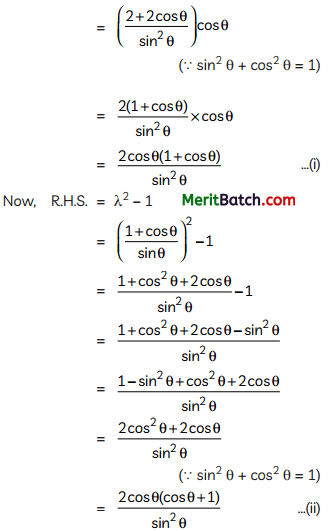From (i) and (ii)
2 + 1) cos θ = λ2 – 1
Hence, Proved.Question 28.
A lot consists of 48 mobile phones of which 42 are good, 3 have only minor defects and 3 have major defects. Varnika will buy a mobile if it is good but the trader will only buy a mobile if it has no major defect. One phone is selected at random from the lot. What is the probability that it is:
(A) acceptable to Varnika?
OR
In the given figure, the radii OA and OB are perpendicular to each other.If PA and PB are tangents to the circle, assign a special name to the quadrilateral OAPB and justify your answer.
Total no. of mobile phones = 48
No. of mobiles which are in good condition = 42
No. of mobiles with minor difects = 3
No. of mobiles with mayor difects = 3
(A) Total no. of mobile phones in good condition = 42
P(mobile phone is acceptable to Varnika) = $$\frac{42}{48}$$
= $$\frac{7}{8}$$

(B) Total no. of mobile phones in good condition and with minor defect = 42 + 3 = 45
P(mobile phone is acceptable to trader) = $$\frac{45}{48}$$
= $$\frac{15}{16}$$

OR

Here, ∠AOB = 90° (given)
and ∠OAP = ∠OBP = 90°
(As tangent is ± to the radius)
∴ ∠APB = 90°
Now, OA = OB (radii of circle)
AP = PB (tangents from an external point are equal)
Since, all the angles are 90° and adjacent sides are equal.
Hence, the quadrilateral OAPB is a square.

Question 29.
An isosceles triangle ABC has been inscribed in a circle in which AB = AC Prove that the tangent at A is parallel to BC
Given: ∆ABC is an isoceles triangle, in which AB = AC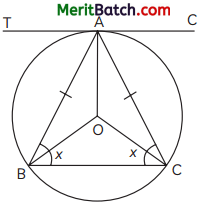To Prove : Tangent at A is parallel to BC
Proof : Since, AB = AC
Let ∠ABC = ∠ACB = x
∵ ∠AOB = 2∠ACB = 2x
(by property)
Now, in ∆AOB,
∠OBA = ∠OAB
(∵ OA = OB, radii of circle)
Now, ∠OBA + ∠OAB + ∠AOB = 180°
(by angle sum property)
∴ ∠OAB + ∠OAB + 2x = 180°
∠OAB = 90° – x ∠TAO = 90°
(As tangent is ⊥ to radius)
∠TAB = ∠TAO – ∠BAO = 90° – (90° – x) = x
∴ ∠TAB = ∠ABC = x
∴ TA || BC
Hence, Proved.

Question 30.
The radius of the circumcircle of a right angle triangle is 3 cm and altitude drawn to the hypotenuse is 2 cm. Rnd the area of the right angled triangle.
In case of right triangle, the hypotenuse is a diameter of the circumcircle, and its centre is exactly at the mid point of the hypotenuse.As radius of its circumcircle is 3cm,
So, AC (hypotenuse) = 3 × 2 = 6 cm
BD = 2 cm
Area of ∆ABC = $$\frac{1}{2}$$ × AC × BD
= $$\frac{1}{2}$$ × 6 × 2 = 6 cm2Question 31.
In a shop an offer is given of colour pencils and crayons of children crayons were being sold in packs of 24 and colours pencils in packs of 32 each. If you want full packs of both and the same number of pencils and crayons, how many of each Sushma need to buy?
OR
Show graphically that the following pair of linear equations has no solution: 2x + 4y = 10; 3x + 6y = 12
We have to find the LCM of number of the crayons in the box and number of colour pencils in each pack which is 24 and 32.
24 = 23 × 3
32 = 25
LCM (24, 32) = 25 × 3 = 96
In 1 pack = 24 crayons
96 crayons = 4 × 24 = 4 packs
32 pencils = 1 packs
96 pencils = 3 × 32 = 3 packs
Hence, Sushma need to buy 4 packs of crayons and 3 packs of colour pencil.
OR
Given, pair of linear equations are:
2x + 4y = 10 …(i)
3x + 6y = 12 …(ii)
For equation (i)
y = $$\frac{10-x}{4}$$

 x 5 1 -1 y 0 2 3

For equation (ii)
y = $$\frac{12-3x}{6}$$

 x 2 4 5 y 1 0 -0.5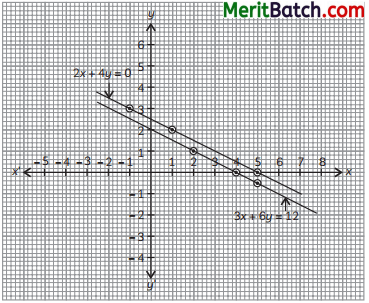Here, the equations represents a pair of parallel lines which do not coincide, so it has no solution.

Section – D
(Section D consists of 4 questions of 5 marks each.)

Question 32.
A motor boat whose speed in still water is 15 km/h. It can go 30 km upstream and return downstream to the original point in 4 hours 30 minutes. Rnd the speed of the stream.
Let the speed of stream be x km/hr
Speed of boat in upstream = (15 – x) km/hr
Speed of boat in downstream = (15 + x) km/hr
ATQ
$$\frac{30}{15-x}+\frac{30}{15+x}=4+\frac{30}{60}$$
⇒ $$30\left[\frac{15+x+15-x}{(15+x)(15-x)}\right]=\frac{9}{2}$$
⇒ $$30\left[\frac{30}{225-x^2}\right]=\frac{9}{2}$$
⇒ $$\frac{100}{225-x^2}=\frac{1}{2}$$
⇒ 225 – x2 = 200
⇒ x2 = 25
⇒ x = ±5
But speed cannot be negative
x = 5
Hence, speed of the stream be 5 km/hr.Question 33.
In a trapezium ABCD with AB || DC O is the point where diagonals interact. Through O, a line segment PQ is drawn parallel to AB which meets AD in P and BC in Q. Now, prove that PO = QO.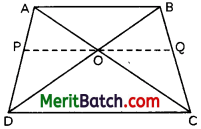OR
If cos θ – sin θ = $$\sqrt{2}$$sin θ. then show tljpt cos θ + sin θ= $$\sqrt{2}$$cos θ.
Given: ABCD is a trapezium AB || CD & PQ || AB
So, PQ || AB || CD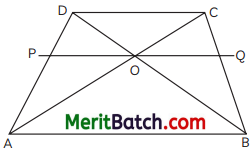Also OP || AB and OQ || AB
To Prove: OP = OQ
OP || AB
∴ $$\frac{\mathrm{AP}}{\mathrm{PD}}=\frac{\mathrm{OA}}{\mathrm{OC}}$$ ……(i) (by BPT)
In ∆ABC
OQ || AB
∴ $$\frac{\mathrm{BQ}}{\mathrm{QC}}=\frac{\mathrm{OA}}{\mathrm{OC}}$$ …..(ii) (by BPT)
From (i) and (ii)
$$\frac{\mathrm{AP}}{\mathrm{PD}}=\frac{\mathrm{BQ}}{\mathrm{QC}}$$OR

Given: cos θ – sin θ = $$\sqrt{2}$$sin θ
On squaring on both sidesQuestion 34.
From the top of a tower 40 m tall, the angle of depression of the nearer of the two points A and B on the ground diametrically opposite sides of the tower is 45°. Show that the angle of depression θ of the other point if the distance of the two points from the base of the tower are in the ratio 1: 2, is given by tan θ = $$\frac{1}{2}$$.
OR
A test tube has a hemisphere-shaped lower portion and a cylindrical upper portion with the same radius. The test tube fills up to the point of being exactly full when 4554/7 cu cm of water is poured, but when only 396 cu cm is added, 9 cm of the tube is left empty. Calculate the test tube’s radius and the cylindrical part’s height. (5)PQ is a tower of height 40 m.
A and B are the points of observation
Given : AQ : QB = 1 : 2
AQ = x and QB = 2x

In ∆APQ,
tan 45° = $$\frac{PQ}{AQ}$$ = $$\frac{40}{x}$$
x = 40 m ……(i)

In ∆PQB,
tan θ = $$\frac{PQ}{BQ}$$ = $$\frac{40}{2x}$$
tan θ = $$\frac{40}{2×40}$$ = $$\frac{1}{2}$$ (from (i))
Hence, Proved.

OR
Volume of water that can fill the test cube = $$\frac{4554}{7}$$ cm3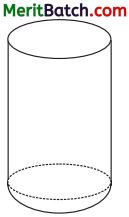Let ‘r be the radius of cylinder and hemispherical part.
And let ‘h’ be height of cylinderical part.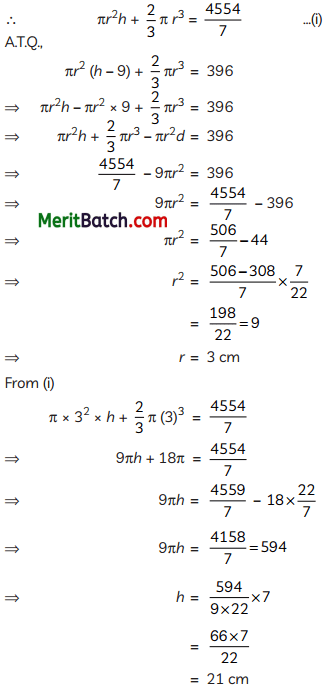Hence, radius of the test tube is 3 cm and height of cylinderical part is 21 cmQuestion 35.
The severe acute respiratory syndrome corona virus type 2 (SARS-CoV-2) is the primary cause of the continuing COVID-19 pandemic, sometimes referred to as the coronavirus pandemic. The age distribution of patients admitted to the hospital for a given day is shown in the following table. Find the median for the following table.

 Class Frequency Less than 20 0 Less than 30 4 Less than 40 16 Less than 50 30 Less than 60 46 Less than 70 66 Less than 80 82 Less than 90 92 Less than 100 100

Converting the given cumulative frequency distribution in continuous class intervals.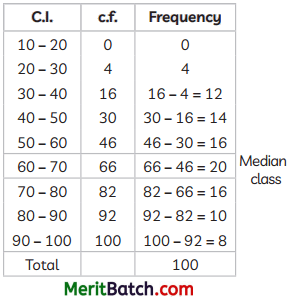Here N = 100
$$\frac{N}{2}$$ = $$\frac{100}{2}$$
Hence, median class is 60 – 70
l = 60, c.f. = 46, f = 20, h = 10
Median = $$l+\left(\frac{\frac{\mathrm{N}}{2}-c f}{f}\right) \times h$$
= $$60+\left(\frac{50-46}{20}\right) \times 10$$
= $$60+\frac{40}{20}$$
= 62
Hence, the median is 62.

Section – E
(Case Study Based Questions)
(Section E consists of 3 questions. All are compulsory.)

Question 36.
Mr. Punit arranged a lunch party for some of his friends. The expenses of the lunch are partly constant and partly proportional to the number of guests. The expense amount for 7 guests is ₹ 650, and ₹ 970 for 11 guests.Let the constant expenses be denoted by ₹ x and proportional expenses per person by ₹ y
Based on this information, answer the following questions:
(A) How the situation stated above will be translated into a pair of linear equation? (1)
(B) What are the proportional expenses per person?
OR
What are the constant expenses? If there would be 15 guests at the lunch party, thus what amount Mr. Punit has to pay? (2)
(C) Find the value of kfor which the system of linear equations x + 2y = 3, 5x + ky + 7 = 0 is inconsistent. (1)
(A) Given, constant expenses be ₹ x and proportional expenses per person be ₹ y
ATQ, x + 7y = 650
x + 11y = 970

(B) The equations in part (A) are
x + 7y = 650 …(i)
x + 11y = 970 …(ii)
On subtracting (i) from (ii)4y = 320
y = 80
OR
Put the value of y = 80 in x + 7y = 650
x + 7 × 80 = 650
x = 650 – 560 = 90
If no of guests are 15, then total expenses = 90 + 80 × 15
90+1200
= 1290

(C) The system of euations will be inconsistent, if
$$\frac{a_1}{a_2}=\frac{b_1}{b_2} \neq \frac{c_1}{c_2}$$
Here, a1 = 1, b1 = 2, c1 = -3
a2 = 5, b2 = k, c2 = k
So, $$\frac{1}{5}=\frac{2}{k} \neq \frac{3}{7}$$
i.e., k = 10Question 37.
To raise social awareness about hazards of smoking, a school decided to start ‘No smoking’ campaign. 10 students are asked to prepare campaign banners in the shape of a triangle. The vertices of one of the triangle are P(-3, 4), Q(3, 4) and R(-2, -1).Based on this information, answer the following questions:
(A) Find the coordinates of centroid of APQR. If U be the mid-point of line joining R and P, then the coordinates of U.
OR
And the coordinates of centroid of ASTU. (2)
(B) If S be the mid-point of line joining P and Q, then find the coordinates of S. (1)
(C) If T be the mid-point of line joining R and Q, then find the coordinates of T. (1)
(A) Coordinates of centroid
= $$\left(\frac{x_1+x_2+x_3}{3}, \frac{y_1+y_2+y_3}{3}\right)$$
= $$\left(\frac{-3+3+(-2)}{3}, \frac{4+4-1}{3}\right)$$
= $$\left(\frac{-2}{3}, \frac{7}{3}\right)$$

Coordinates of mid-point U(x,y)
= $$\left(\frac{-3-2}{2}, \frac{4-1}{2}\right)$$
= $$\left(\frac{-5}{2}, \frac{3}{2}\right)$$

OR

Coordinates of S(0, 4)

Coordinates of T$$\left(\frac{1}{2}, \frac{3}{2}\right)$$
Cordinates of U$$\left(\frac{-5}{2}, \frac{3}{2}\right)$$
Coordinates of ∆STU
= $$\left[\frac{0+\frac{1}{2}-\frac{5}{2}}{3}, \frac{4+\frac{3}{2}+\frac{3}{2}}{3}\right]$$
= $$\left(\frac{-2}{3}, \frac{7}{3}\right)$$

(B) Coordinates of S(x, y)
= $$\left(\frac{-3+3}{2}, \frac{4+4}{2}\right)$$
= (0, 4)

(C) Coordinates of mid point T(x, y)
= $$\left(\frac{3-2}{2}, \frac{4-1}{2}\right)$$
= $$\left(\frac{1}{2}, \frac{3}{2}\right)$$Question 38.
Joginder a carpenter designs a trapezium ABCD shaded model as shown in the picture below in which AB // DC and diagonals AC and BD divides each other in the ratio 1 : 3. Later he find that the side AB is 3 times the side CD. After that he designed various other trapezium shaped model with some other measurements as mentioned in some questions given below. Help him to determine the measurements asked for.Based on this information, answer the following questions:
(A) In the above model, if OA = x + 15, OB = x – 7, OC = x – 12, OD = x – 2, then what is the value of x ?
OR
In the trapezium model shown, AB || EF || DC. Then, find AE × FC(B) In the trapezium model shown, find AP × DP.(C) In the quadrilateral model shown, ∠1 = ∠3, ∠2 = ∠4
If $$\frac{EC}{AE}=\frac{DE}{BE}=\frac{1}{2}$$ and DC = 4 cm, then, find AB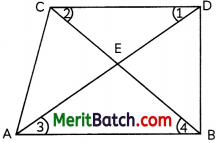Given: $$\frac{AB}{CD}$$ = $$\frac{3}{1}$$ and AB || CD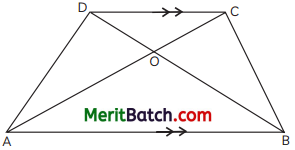(A) Given: OA = x + 15, OB = x – 7, OC = x – 12, OD = x – 2
In ∆AOB and ∆DOC
∠OBA =∠ODC
(alternate pair of angles)
∠OAB = ∠OCD
(alternate pair of angles)
∆AOB ~ ∆COD
$$\frac{O A}{O C}=\frac{O B}{O D}=\frac{A B}{C D}$$
(corresponding sides of similar ∆s)
$$\frac{x +15}{x – 12}=\frac{x – 7}{x – 2}$$
⇒ (x + 15) (x – 2) = (x – 12) (x – 7)
⇒ x2 + 15x – 2x – 30 = x2 – 12x – 7x + 84
⇒ 13x – 30 = – 19x + 84
⇒ 13x + 19x = 84 + 30
⇒ 32x = 114
⇒ x = $$\frac{114}{32}=\frac{57}{16}$$
OR
Here, AB || EF || CD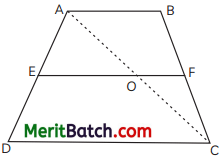∠PAB = ∠PCB
(alternate pair of angles)∠PBA = ∠PDC
(alternate pair of angles)
∆APB ~ ∆CPD
$$\frac{AP}{PC}$$ = $$\frac{BP}{PD}$$
⇒ AP × PD = BP × PC

(C) Here ∠1 = ∠3, ∠2 = ∠4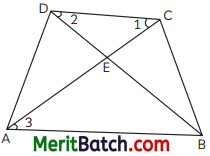In ∆DEC and ∆AEB
∠1 = ∠3
∠2 = ∠4
∆DEC ~ ∆AEB
⇒ $$\frac{AE}{EC}$$ = $$\frac{BE}{DE}$$ = $$\frac{AB}{CD}$$
(by property of similar triangles)
$$\frac{AB}{CD}$$ = $$\frac{2}{1}$$
⇒ $$\frac{AB}{4}$$ = $$\frac{2}{1}$$ ⇒ AB = 8 cm